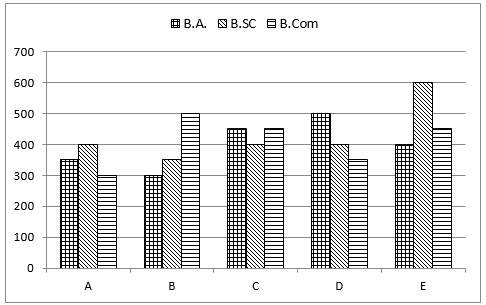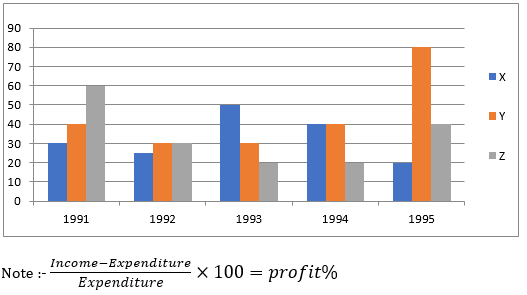IBPS RRB Quantitative Aptitude Quiz

Here, we are providing IBPS RRB Study Plan as there is left not enough time to deal in details. The questions asked in the quantitative aptitude section are calculative and very time-consuming. But once dealt with proper strategy, speed, and accuracy, this section can get you the maximum marks in the examination. Following is the Quantitative Aptitude Quiz to help you practice with the best of latest pattern questions.

Directions (1-5): Study the following graph carefully to answer the questions that follow:
Number of Girls and Boys participating in a rally from five different schoolsQ1. What is the total number of girls participating in the rally from schools A and C together?

825
875
950
975
925

Q2. The number of boys participating in the rally from school B is what percent of the total number of students participating in the rally from that school? (Rounded off to two digits after decimal.)
48.84 %
47.37 %
49.28 %
46.46 %
47.98%

Q3. The number of girls participating in the rally from School E is approximately what percent of the number of boys participating in the rally from the same school?
81 %
106 %
122 %
98 %
114 %

Q4. What is the respective ratio of total number of girls participating in the rally from schools D and C together to the total number of boys participating in the rally from schools A and B together?
23 : 18
35 : 43
41 : 38
20 : 21
39 : 40
Solution:
Total number of girls participating in the rally from schools D and C together = 475 + 500 =975
Total number of boys participating in the rally from schools A and B together = 475 + 525 = 1000
Required ratio= 975: 1000 = 39: 40

Q5. What is the average number of girls participating in the rally from all schools together?
500
460
525
505
650

Directions (6-10): Study the following bar graph and answer the questions that follow.
The following bar graph shows the total no. of students (on vertical axes) in five different colleges (on horizontal axes) studying in three different streams.Q6. If 140/9% of students in B.A. disciplines in college C, are females, then number of males in B.A. disciplines in college C are approximately what percent of females in college D for B.A discipline if no. of females in B.A. discipline in college D are 2000/7% more than females in B.A. discipline of College C.

110%
90%
80%
140%
130%

Q7. Number of students in B.SC from college C and E together are approximately what percent of the total number of students in B. Com from all colleges together?
42%
32%
49%
38%
28%

Q8. What is the ratio of 2/5 of students in college A and C together to 5/3 of students in college D & E together.
47 : 225
80 : 123
43 : 223
31 : 117
None of these

Q9. If 76/5% of students in college D, leaves the college and number of students from all three discipline (i.e. B.A., B Sc, B.Com) who leave college D are in the ratio 8 : 6 : 5 respectively, then remaining student from B. com in college D are what % of student from B.Sc discipline from college E?
48%
50%
43%
52%
49%

Q10. Total students from B.A. discipline from all colleges together are approximately what % more or less than total students from B.Sc discipline from all colleges together?
9%
7%
5%
11%
13%

Directions (11-15): The following chart represent the Percentage of profit by three retailers X, Y and Z in given five years.Q11. If Income of X in 1992 is equal to expenditure of Y in 1994, what is the profit of X in 1992 if income of Y in 1994 is Rs. 7 Lac.

2,00,000
3,00,000
1,00,000
1,54,000
None of these

Q12. What is the average income of X in all these years if expenditure of X in 1991 is Rs 1 Lac and it increases by Rs. 50,000 every year?
2.215 lacs
2.655 lacs
3.412 lacs
2.913 lacs
1.817 lacs

Q13. In 1995, expenditure of X, Y and Z are in ratio 2:3:5. What will be the ratio of their profits?
1:6:5
2:5:6
1:3:2
2:3:2
None of the above

Q14. Income of X in 1993 is Rs. 4,00,000 which is 20% less than the expenditure of Z in the same year. What is the difference between their profits? (in approximate)
Rs. 45,333
Rs. 15,050
Rs. 50,000
Rs. 40,667
Rs. 33,333

Q15. In which of the following two years, the average of profit percent of all three together are equal.?
1992 & 1993
1993 & 1994
1992 & 1994
1991 & 1992
1992 & 1995

You May also like to Read: